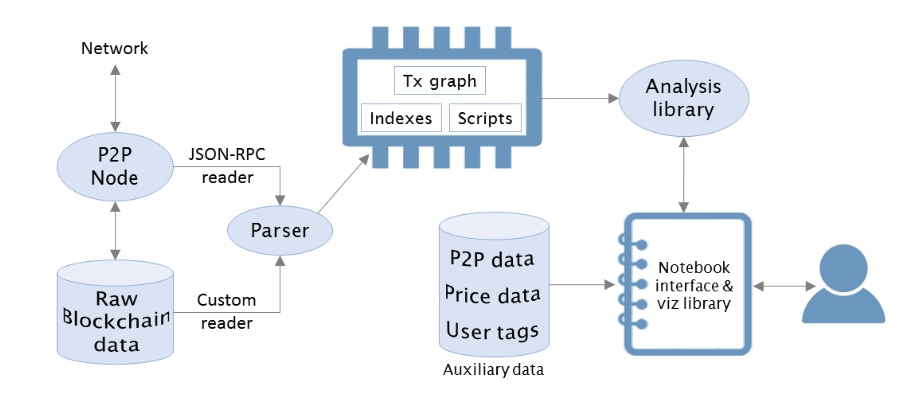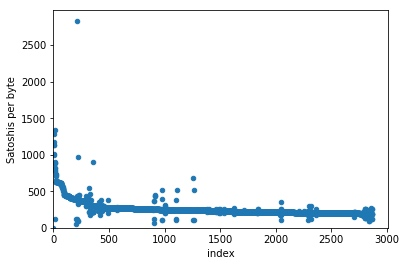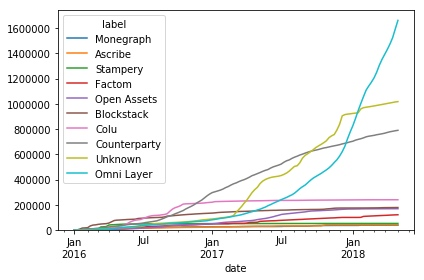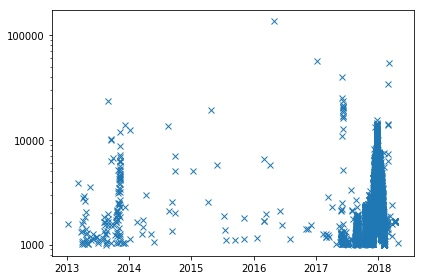# 区块链数据分析框架-BlockSci

https://www.interchains.cc/21604.html

### 区块链blockchain数据分析框架-BlockSci

• BTC
• 数据分析

BTC以太坊eth这样的区块链blockchain中包含了大量的数据，其中蕴藏着区块链blockchain生态系统中用户、企业和矿工的行为模式等重要信息。BlockSci是用C++开发的高性能的区块链blockchain数据分析框架，它可以帮助我们快速分析BTC区块链blockchain的数据。本文将介绍BlockSci的安装方法，及如何利用BlockSci进行区块链blockchain数据分析。

## 安装BlockSci框架

BlockSci的主要开发语言是C++，本地编译需要GCC 7.2+和CLang 5+。 官方文档仅提供了Ubuntu 16.04和Mac OS10.13下的本地安装方法， 其他系统可以尝试本地安装或使用官方提供的AWS EC2镜像。

## BlockSci框架概述

BlockSci包含两个主要的组件：解析器和分析库。

• 解析器用来解析区块链blockchain数据并转换为便于执行分析任务的BlockSci数据格式。
• 分析库则用来编写数据分析任务。区块链blockchain数据分析框架-BlockSci” />

## BlockSci解析器 / Parser

BlockSci的解析器支持两种处理原始区块链blockchain数据的机制：磁盘模式和RPC模式。

• 磁盘模式是处理BTC区块数据的推荐模式，它可以直接读取并解析磁盘上的BTC区块数据文件，因此速度要快得多。但是这也意味着磁盘模式不能支持更多的区块链blockchain，因为不同的区块链blockchain的磁盘数据文件格式不同。

使用以下命令以磁盘模式解析BTC区块数据：

``blocksci_parser --output-directory bitcoin-data update disk --coin-directory .bitcoin``

参数说明：

• –output-directory：解析结果输出目录
• –coin-directory：bitcoin core数据目录
• RPC模式使用数据加密货币区块链blockchain节点的RPC接口来提取数据，而RPC接口是大多数（山寨币）区块链blockchain都支持的与BTC类似的二次开发接口（虽然优势也有一些差异），因此在BTC之外的区块链blockchain数据解析，BlockSci通常采用RPC模式，例如对于Zcash和Namecoin。

要使用RPC模式的BlockSci解析器，需要一个区块链blockchain全节点并且启用txindex选项。 命令格式如下：

``blocksci_parser --output-directory bitcoin-data update rpc --username [user] --password [pass] --address [ip] --port [port]``

参数说明：

• –output-directory：解析结果输出目录
• –port：RPC API监听端口

## BlockSci分析库 / Analysis Library

``#include &lt;blocksci/blocksci.hpp>  int main(int argc, const char * argv[]) {   blocksci::Blockchain chain{"file_path_to_output-directory"}; }``

BlockSci目前仅支持Python 3，使用方法很简单：导入BlockSci库，然后利用解析器输出目录的数据构建Blockchain对象：

``import blocksci chain = blocksci.Blockchain("file_path_to_parser_output-directory")``

## BlockSci区块链blockchain数据分析示例

``import blocksci import matplotlib.pyplot as plt import matplotlib.ticker import collections import pandas as pd import numpy as np  chain = blocksci.Blockchain('your_parser_data_directory')``

### BTC地址类型使用情况分类统计

``net_coins_per_block = chain.map_blocks(lambda block: block.net_address_type_value())  df = pd.DataFrame(net_coins_per_block).fillna(0).cumsum()/1e8 df = chain.heights_to_dates(df) df = df.rename(columns={t:str(t) for t in df.columns}) ax = df.resample("W").mean().plot() ax.set_ylim(ymin=0)``

### BTC单区块交易手续费率可视化分析

``example_block_height = 465100 df = pd.DataFrame(chain[example_block_height].txes.fee_per_byte(), columns=["Satoshis per byte"]) ax = df.reset_index().plot.scatter(x="index", y="Satoshis per byte") ax.set_ylim(0) ax.set_xlim(0) plt.show()``区块链blockchain数据分析框架-BlockSci” />

### BTC二层协议使用情况可视化分析

BTC交易内可以嵌入二层协议以实现对其他应用的支持，例如omni layer 的usdt代币就是最常见的一种BTC二层协议应用。下面的代码使用BlockSci的区块链blockchain对象的`blocks.txes`属性提取在交易输出中包含OP_RETURN脚本的交易，并显示二层协议的使用情况。

``txes = chain.blocks.txes.including_output_of_type(blocksci.address_type.nulldata).all labels = [(tx.block.time, blocksci.label_application(tx)) for tx in txes]  df = pd.DataFrame(labels, columns=["date", "label"]) df = df.reset_index().groupby(["date", "label"]).count().unstack(level=-1).fillna(0) df.columns = df.columns.droplevel() important_columns = list(df[df.index > pd.to_datetime("1-1-2016")].sum().sort_values()[-10:].index) important_columns = [x for x in important_columns if "Address" not in x] ax = df[df.index > pd.to_datetime("1-1-2016")].cumsum().resample("w").mean()[important_columns].plot() ax.set_ylim(0) plt.tight_layout()``区块链blockchain数据分析框架-BlockSci” />

### BTC2017年各月平均交易手续费可视化分析

``converter = blocksci.CurrencyConverter() blocks = chain.range('2017') times = blocks.time  df = pd.DataFrame({"Fee":fees}, index=times) df = converter.satoshi_to_currency_df(df, chain) ax = df.resample("d").mean().plot(legend=False) ax.set_ylim(ymin=0) plt.tight_layout()``

### BTC大额手续费交易可视化分析

``high_fee_txes = chain.cpp.filter_tx("fee(tx) > 10000000", 0, len(chain)) converter = blocksci.CurrencyConverter()  df = pd.DataFrame([(tx.block.height, tx.fee) for tx in high_fee_txes], columns=["height", "fee"]) df.index = df["height"] df["fee"] = df.apply(lambda x: converter.satoshi_to_currency(x["fee"], chain[x["height"]].time), axis=1) df = df[df["fee"] > 1000] df = chain.heights_to_dates(df) fig, ax = plt.subplots() ax.plot_date(df.index, df["fee"], fmt="x") ax.set_yscale("log") formatter = matplotlib.ticker.ScalarFormatter(useOffset=False) formatter.set_scientific(False) ax.yaxis.set_major_formatter(formatter) plt.tight_layout()``区块链blockchain数据分析框架-BlockSci” />

2017年超过1000美元手续费的交易激增，从一个侧面说明了这一年涌入了大量数字加密货币的新手。